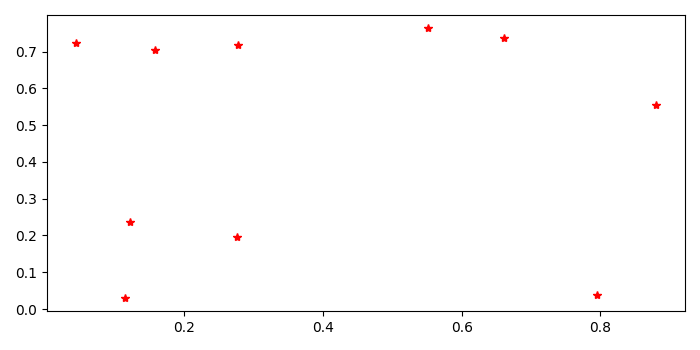# How to get pixel coordinates for Matplotlib-generated scatterplot?

To get pixel coordinates for matplotlib-generated scatterplot, we can take the following steps −

• Set the figure size and adjust the padding between and around the subplots.
• Initialize a variable "n" to hold the number of sample data.
• Create a figure and a set of subplots.
• Make a scatter plot.
• Get the x and y data points using get_data() method.
• Get the pixel value of the plot.
• Get the pixel tranformed data.
• Get the figure width and height in points or pixels
• Print the x and y pixels value.
• To display the figure, use show() method.

## Example

import numpy as np
import matplotlib.pyplot as plt

plt.rcParams["figure.figsize"] = [7.00, 3.50]
plt.rcParams["figure.autolayout"] = True

n = 10
fig, ax = plt.subplots()

points, = ax.plot(np.random.random(n), np.random.random(n), 'r*')
x, y = points.get_data()

pixels = ax.transData.transform(np.vstack([x, y]).T)
x, y = pixels.T

width, height = fig.canvas.get_width_height()
y = height - y

print("The pixel coordinates are: ")
for xp, yp in zip(x, y):
print('{x:0.2f}\t{y:0.2f}'.format(x=xp, y=yp))

plt.show()

## Output

It will produce the following outputIt will also print the pixel coordinates of the matplotlib-generated scatterplot on the console

The pixel coordinates are:
564.93   161.83
446.27   112.60
153.39   247.65
236.90   258.34
519.10   301.04
237.66   118.16
149.71   303.29
386.74   105.81
172.93   121.81
110.94   116.20